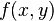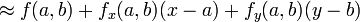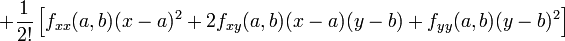# Weird mathematica problem

• Mathematica
does anyone know how to derive multivariate taylor series in mathematica? by default it is computed in a very strange: "Series performs a series expansion successively with respect to each variable. The result in this case is a series in x, whose coefficients are series in y. "

What don't you like about this representation? If you want to have the terms ordered by the total degree of the monomials, there seems not to be a built-in way to do it. (But it shouldn't be too hard to come up with a work-around oneself ...)

Try using the function Collect:

Collect[Series[Exp[x y], {x, 0, 8}, {y, 0, 8}] , {x, y}]

If that's not what you want, then I fail to understand. I'm sure there must be a way to do what you are asking, however.

Now I understand the problem. That's strange that Mathematica does not have much material about multivariate series, so we can add it ourselves.

Code:
multiVarSeries[f_, x_List, a_List, k_Integer] :=  Block[{n, F},
Evaluate[Fold[Sum,
Product[(1/n[i]!) (x[[i]] - a[[i]])^(n[i]), {i, 1, Length[x]}]
((Fold[D, F@@x, Table[{x[[i]], n[i]}, {i, 1, Length[x]}]]) /.
Table[x[[i]] -> a[[i]], {i, 1, Length[x]}]),
Table[{n[i], 1, k}, {i, 1, Length[x]}]]] /. F -> Function[x, f]]

Copy and paste that function into a new cell and then execute it with shift + enter. After that you can invoke the function. Here is a simple example:

multiVarSeries[Exp[x y], {x, y}, {0,0}, 2]

This says to expand the function Exp[x y] with respect to the variables x and y around the point {0,0} up to order 2 (in both variables, I don't let you specify the order separately for each individual variable). The output is of course:

$$\frac{x^3 y^3}{6}+\frac{x^2 y^2}{2}+x y$$

i don't know much about mathematica programming so can you adjust your function so that it computes to a total order of n? e.g. for order 2 xy terms are written out and x^2 and y^2 terms but not x*y^2. and you example seems to show terms up to order 3

ps
how can i learn to program mathematica

i don't know much about mathematica programming so can you adjust your function so that it computes to a total order of n? e.g. for order 2 xy terms are written out and x^2 and y^2 terms but not x*y^2. and you example seems to show terms up to order

Yes, I understand your complaint with the function. One thing you can do is make n larger than you need and then use a filter to get only the terms you want. It is more work than it is worth for me to change the function to match that behavior.

how can i learn to program mathematica

There is no good way. If you are really wealthy, you can do workshops online with Wolfram Inc that will teach you how to program Mathematica. Otherwise you have to do what I did, which is to read the built-in help and practice for months.

well it doesn't work. i read on a grou somewhere the Normal[Series[Exp[x*t + y*t], {t, 0, 2}]] /. t -> 1 would work and it does but it doesn't work for my function. any ideas?

well it doesn't work. i read on a grou somewhere the Normal[Series[Exp[x*t + y*t], {t, 0, 2}]] /. t -> 1 would work and it does but it doesn't work for my function. any ideas?

What doesn't work and what function are you trying to do this with?

so here i am again with the same problem. how in the heck do i get mathematica to give me this representation:of a function expanded to second order in both of its arguments

Last edited by a moderator: